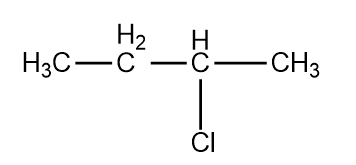When CH3CH2CHCl2 is treated with NaNH2, the product formed is

1. CH3-CH=CH2

2. CH3-C$\equiv$CH

3. CH3CH2CH${<}_{{\mathrm{NH}}_{2}}^{{\mathrm{NH}}_{2}}$

4. CH3CH2C${<}_{{\mathrm{NH}}_{2}}^{\mathrm{Cl}}$

Subtopic:  Physical Properties |
To view explanation, please take trial in the course below.
NEET 2022 - Target Batch - Aryan Raj Singh
To view explanation, please take trial in the course below.
NEET 2022 - Target Batch - Aryan Raj Singh

Difficulty Level:

Chlorobenzene reacts with Mg in dry ether to give a compound (A),

'A' further reacts with ethanol to yield -

1. Phenol

2. Benzene

3. Ethylbenzene

4. Phenylether

Subtopic:  Mechanism of Reactions |
To view explanation, please take trial in the course below.
NEET 2022 - Target Batch - Aryan Raj Singh
To view explanation, please take trial in the course below.
NEET 2022 - Target Batch - Aryan Raj Singh

Difficulty Level:

R-CH2-CCl2-R $\stackrel{\mathrm{Reagent}}{\to }$R-C$\equiv$C-R. The reagent is

1. Na

2. HCl in H2O

3. KOH in C2H5OH

4. Zn in alcohol

Subtopic:  Physical Properties |
To view explanation, please take trial in the course below.
NEET 2022 - Target Batch - Aryan Raj Singh
To view explanation, please take trial in the course below.
NEET 2022 - Target Batch - Aryan Raj Singh

Difficulty Level:

Ethyl chloride is converted into diethyl ether by -

1. Wurtz reaction
2. Grignard reaction
3. Perkin's reaction
4. Williamson's synthesis

Subtopic:  Mechanism of Reactions |
To view explanation, please take trial in the course below.
NEET 2022 - Target Batch - Aryan Raj Singh
To view explanation, please take trial in the course below.
NEET 2022 - Target Batch - Aryan Raj Singh

Difficulty Level:

The alkyl halide is converted into an alcohol by

3. Dehydrohalogenation    4. Elimination

Subtopic:  Physical Properties |
To view explanation, please take trial in the course below.
NEET 2022 - Target Batch - Aryan Raj Singh
To view explanation, please take trial in the course below.
NEET 2022 - Target Batch - Aryan Raj Singh

Difficulty Level:

The addition of Br2 on cis-but-2-ene gives:

1. Racemic mixture of 2,3-Dibromobutane

2. Meso form of 2,3-Dibromobutane

3. Dextro form of 2,3-Dibromobutane

4. Laevo form of 2,3-Dibromobutane

Subtopic:  Isomerism & Chirality |
To view explanation, please take trial in the course below.
NEET 2022 - Target Batch - Aryan Raj Singh

Difficulty Level:

The number of different substitution products possible when ethane is allowed to react with bromine in sunlight are:

1. 9

2. 6

3. 8

4. 5

Subtopic:  Chemical Properties | Isomerism & Chirality |
To view explanation, please take trial in the course below.
NEET 2022 - Target Batch - Aryan Raj Singh
To view explanation, please take trial in the course below.
NEET 2022 - Target Batch - Aryan Raj Singh

Difficulty Level:, Cl obtained by chlorination of n-butane, will be

1. meso form

2. racemic mixture

3. d-form

4. l-form

Subtopic:  Mechanism of Reactions |
To view explanation, please take trial in the course below.
NEET 2022 - Target Batch - Aryan Raj Singh
To view explanation, please take trial in the course below.
NEET 2022 - Target Batch - Aryan Raj Singh

Difficulty Level:

The reactivity order of halides for dehydrohalogenation is

1. R-F>R-Cl>R-Br>R-I

2. R-I>R-Br>R-Cl>R-F

3. R-I>R-Cl>R-Br>R-F

4.  R-F>R-I>R-Br>R-Cl

Subtopic:  Chemical Properties |
To view explanation, please take trial in the course below.
NEET 2022 - Target Batch - Aryan Raj Singh
To view explanation, please take trial in the course below.
NEET 2022 - Target Batch - Aryan Raj Singh

Difficulty Level:

In ${\mathrm{S}}_{{\mathrm{N}}^{2}}$ reactions, the correct order of reactivity for the following compounds : ${\mathrm{CH}}_{3}\mathrm{Cl}$${\mathrm{CH}}_{3}{\mathrm{CH}}_{2}\mathrm{Cl}$${\left({\mathrm{CH}}_{3}\right)}_{2}\mathrm{CHCl}$ and ${\left({\mathrm{CH}}_{3}\right)}_{3}\mathrm{CCl}$ is :

1. ${\mathrm{CH}}_{3}\mathrm{Cl}$>${\left({\mathrm{CH}}_{3}\right)}_{2}\mathrm{CHCl}$>${\mathrm{CH}}_{3}{\mathrm{CH}}_{2}\mathrm{Cl}$>${\left({\mathrm{CH}}_{3}\right)}_{3}\mathrm{CCl}$

2. ${\mathrm{CH}}_{3}\mathrm{Cl}$>${\mathrm{CH}}_{3}{\mathrm{CH}}_{2}\mathrm{Cl}$>${\left({\mathrm{CH}}_{3}\right)}_{2}\mathrm{CHCl}$>${\left({\mathrm{CH}}_{3}\right)}_{3}\mathrm{CCl}$

3. ${\mathrm{CH}}_{3}{\mathrm{CH}}_{2}\mathrm{Cl}$>${\mathrm{CH}}_{3}\mathrm{Cl}$>${\left({\mathrm{CH}}_{3}\right)}_{2}\mathrm{CHCl}$>${\left({\mathrm{CH}}_{3}\right)}_{3}\mathrm{CCl}$

4. ${\left({\mathrm{CH}}_{3}\right)}_{2}\mathrm{CHCl}$>${\mathrm{CH}}_{3}{\mathrm{CH}}_{2}\mathrm{Cl}$>${\mathrm{CH}}_{3}\mathrm{Cl}$>${\left({\mathrm{CH}}_{3}\right)}_{3}\mathrm{CCl}$

Subtopic:  Mechanism of Reactions |
To view explanation, please take trial in the course below.
NEET 2022 - Target Batch - Aryan Raj Singh
To view explanation, please take trial in the course below.
NEET 2022 - Target Batch - Aryan Raj Singh

Difficulty Level: